# MCAT Physical : Electron Configuration

## Example Questions

← Previous 1

### Example Question #1 : Electron Configuration

What is the electron configuration of potassium after it obtains a +1 charge?

[Ar]4s23d4

[Ar]4s2

[Ar]4s1

[Ne]3s1

[Ar]

[Ar]

Explanation:

Potassium (K) is orignially in the electron configuration of [Ar]4s1. To obtain a +1 charge it loses an electron, resulting in a configuration of [Ar].

### Example Question #2 : Electron Configuration

Which of the following species is represented by the given electron configuration?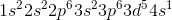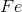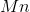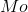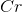Explanation:

Due to the phenomenon of half-orbital stability in the transition metals, electrons can easily move between 4s and 3d orbitals. The atom achieves greater stability from having only one atom in the 4s orbital, allowing a half-filled 3d orbital, as opposed to a full 4s orbital and four electrons in the 3d subshell.

For elements like chromium and copper, which could have valence shell configurations of 4s23d4 and 4s23d9, respectively, an electron from the 4s orbital jumps down to the 3d orbital to harness added stability from the half-filled orbital. The given electron configuration is that of chromium.

Note that you can also solve this question by counting the electrons to determine the atomic number. In this case, the electrons add up to 24, indicating the twenty-fourth element: chromium.

### Example Question #1 : Electron Configuration

What is the correct electronic structure for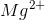?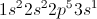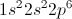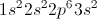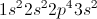Explanation:

Were this question asking for the electronic structure of magnesium (Mg) in its ground state,would be the correct answer; however, the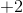charge onmeans that the molecule shed two valence electrons to achieve a more stable orbital. Those electrons will be shed from the outermost valence shell, which in this case is the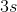shell; therefore,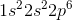is correct.

Note that the ground state of magnesium will have twelve electrons (the same as its atomic number), while the ion will have ten.

### Example Question #4 : Electron Configuration

An oxygen atom and a fluorine atom have the same electron configuration as neon. Which of the following is true about these two atoms?

Oxygen has half the charge of fluorine because it gains half the amount of electrons

Fluorine has half the charge of oxygen because it gains half the amount of electrons

Both atoms have the same charge because they both gain the same amount of electrons

Both atoms are classified as halogens

Fluorine has half the charge of oxygen because it gains half the amount of electrons

Explanation:

The question states that the oxygen and fluorine atoms have the same electron configuration as neon. The electron configuration for neon is, with ten total electrons and eight valence electrons. To match this configuration, both the oxygen and the fluorine atom must have eight valence electrons (two in the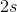orbital and six in the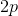orbital).

Recall that atoms in the oxygen group have six valence electrons, whereas halogens have seven valence electrons; therefore, oxygen has six and fluorine has seven valence electrons. To reach a total of eight valence electrons (octet) the oxygen atom must have gained two electrons, giving it a charge of. Similarly, fluorine must have gained one electron, giving it a charge of. Fluorine has half the of charge of oxygen because it gained half the amount of electrons to complete its octet.

### Example Question #1 : Electron Configuration

Which of the following is true about gaining and losing electrons in cobalt?

In cobalt,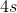is the last orbital that gains electrons and the first orbital that loses electrons

In cobalt,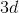is the last orbital that gains electrons and the first orbital that loses electrons

The last orbital that gains electrons in cobalt isand the first orbital that loses electrons in cobalt isThe last orbital that gains electrons in cobalt isand the first orbital that loses electrons in cobalt isThe last orbital that gains electrons in cobalt isand the first orbital that loses electrons in cobalt isExplanation:

Cobalt is a transition metal; therefore, it is found in the D block of the periodic table. A ground state cobalt atom has an electron configuration of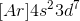. The last orbitals that gain or lose electrons must be either theororbitals, since these are the orbitals with highest energy and located farthest from the nucleus.

Recall that electrons are filled from orbitals of low energy to high energy. Aorbital has a lower energy than aorbital. This means that when you are filling electrons, the last orbital you fill is theorbital. When you are assigning electrons to each orbital you assign two electrons to theorbital and then the remaining seven electrons to the fiveorbitals; therefore,orbitals are filled last when gaining electrons.

When an element loses electrons, the first orbital that loses electrons is the outermost orbital. This occurs because the attractive force of the nucleus on the electron will be weakest in the outermost orbital (because it is farthest away from nucleus); therefore, it will be easy to pull the electron away from the nucleus. In cobalt, the outermost orbital is theorbital (because it has the highest shell number). This means that electrons will be lost from theorbital before theorbital.

Notice that the orbital that gains electron last is not the same orbital that loses electrons first. Gaining electrons is dependent on the energy of the orbital, and losing electrons is dependent on the location of the orbital. The highest energy orbital will gain electron last and the outermost orbital will lose electron first.

### Example Question #6 : Electron Configuration

A certain transition metal has an electron configuration such that itsorbital only has one electron. What would be a valid conclusion about this element?

This is an invalid electron configuration becauseorbitals always contain two electrons in transition metals

The identity of the element is chromium and it contains one electron in each of itsorbitals

The identity of the element is manganese and it contains one electron in each of itsorbitals

The identity of the element is manganese and it contains an emptyorbital

The identity of the element is chromium and it contains one electron in each of itsorbitals

Explanation:

The question states that the element is a transition element and it only has one electron in itsorbital. Recall that transition metals usually have two electrons in itsorbital; however, some transition metals lose one of the electrons from theirorbital and move it to one of theirorbital. This occurs because of a phenomenon called half-shell stability. Half-shell stability states that an element is more stable when all the orbitals are half filled.

This is best exemplified by the transition metal chromium. The electron configuration of chromium, conventionally, would be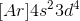. This means that chromium has two electrons in theorbital, one electron in fourorbitals, and an emptyorbital. Half-shell stability states that chromium is more stable if all fiveorbitals have an electron, rather than having an emptyorbital; therefore, an electron from theorbital will be moved to the emptyorbital to fulfill half-shell stability. This gives one electron in theorbital and one electron in each of the fiveorbitals.

Notice that half-shell stability is also observed in molybdenum () and tungsten (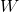). Molybdenum will have one electron in its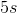orbital and one electron in each of its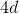orbitals, and tungsten will have one electron in its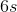orbital and one electron in each of its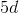orbitals.

### Example Question #1 : Electron Configuration

Which element has the most valence electrons?

Mg

Cu

Zn

As

Ca

As

Explanation:

Valence electrons will be housed in the outer shell (highest numbered orbital) of the electron configuration.

Calcium: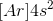Magnesium: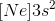Zinc: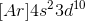Copper: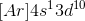Arsenic: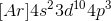Calcium, magnesium, and zinc all have two valence electrons in their highest energy orbital. Copper has only one valence electron. Arsenic has five total valence electrons in the fourth shell.

### Example Question #8 : Electron Configuration

What is the correct electron configuration of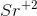?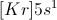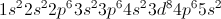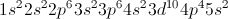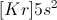Explanation:

An atom that has a charge ofhas lost two electrons. The two lost are always the valence electrons, which are most easily taken from the molecule. Strontium has a normal electron configuration with 38 electrons. In shorthand notation, this would be: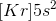Losing two valence electrons will remove the 5s electrons. This leave the configuration as: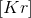This makes sense because krypton has a stable valence octet. Strontium is stable as an ion, meaning that it will also have an octet, allowing it to match the configuration for krypton.

### Example Question #1 : Electron Configuration

Which two elements have the same number of electrons in the 3d shell?

Mn and Fe

Ni and Cu

Cr and Mn

Co and Ni

Cr and V

Cr and Mn

Explanation:

Valence electrons in the d subshell can be odd because a half-filled d orbital is more stable than one with three or four electrons. While strontium, titanium, and vanadium have two electrons in their 4s orbitals, chromium has one in the 4s shell and puts five in the 3d shell.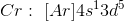This creates a half-filled 4s and 3d shells, which are more stable than a full 4s shell and partial 3d shell. Chromium and manganese thus have the same number of 3d electrons: five.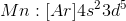### Example Question #10 : Electron Configuration

An atom with the electron configuration 1s22s22p6 could be any of the following except _________.

Na

Mg2+

F-

Ne